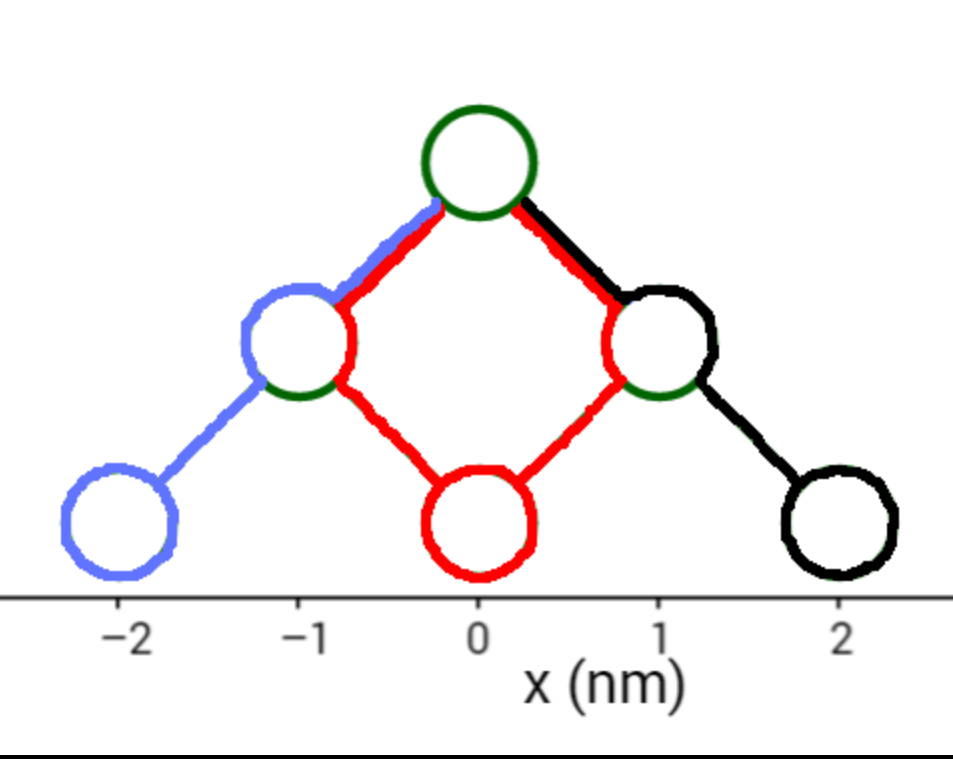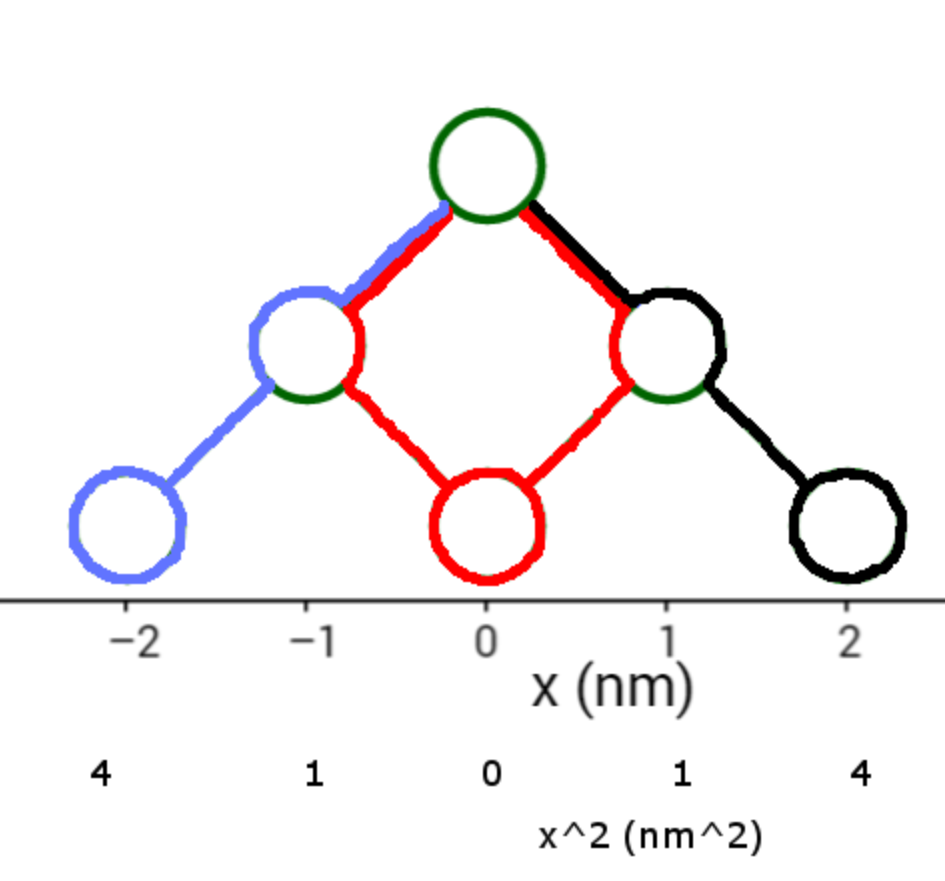• If you are citizen of an European Union member nation, you may not use this service unless you are at least 16 years old.

• Get control of your email attachments. Connect all your Gmail accounts and in less than 2 minutes, Dokkio will automatically organize your file attachments. You can also connect Dokkio to Drive, Dropbox, and Slack. Sign up for free.

View

# Expectation Values via Counting Paths

last edited by 2 years, 8 months ago

We often write quantities likeand. This page explains what these mean and how to find them in the context of a random walk.

prerequisite: Random Walks and Counting Paths

Suppose a large number of molecules all start at zero, then each take a step of length 1 nm, going either left or right with equal probability. This is the beginning of a random walk. Half the molecules go left and half right, which means that on average, their displacement is zero.

This isn't the same as saying that they don't go anywhere - they all moved 1 nm, but if we count moving to the right as positive and moving to the left as negative, then their displacements add up to zero. This is what we mean when we writeThat's not a satisfactory description of the process though. Suppose we let the particles take 1000 random left or right steps. Then they will be spread out quite a bit, even thoughwill still be zero (because at every step, still half move left and half right.)

If the average displacement doesn't tell us about the spread of the molecules, we should find some other description which does. There are different ways to do this. For example, we could find the absolute value of the displacement. Because the absolute value is always positive, it won't be zero on average, and it would give us some real information about how spread out the particles are.

It turns out absolute values are mathematically difficult to work with when following a large bunch of molecules moving randomly. Something that works better is the square of the position.

So imagine that 400 particles start at zero and take two random 1 nm steps. We saw this before in Random Walks and Counting Paths.There is 1 path that leads to -2 nm and 4 total paths, so 1/4 of the particles, or 100, will wind up at -2 nm. 200 will wind up at 0, and 100 at 2 nm.

This is not an exact count; if we really run the experiment, it could be 91, 199, and 110 particles, or some other numbers close to that. But if we did it many times over, it would come out on average to 100 for x = -2 nm, 200 for x = 0 nm, 100 for x = 2nm.

If we then calculate the average of x, or expectation value of x (written), we getThis is from the definition of an average - add up the value of x for each particle, then divide by the total number of particles

Next we'll look at the square of the position, x2. We can start by writing it out underneath x itselfThen the average isHere is another way to think about it. Suppose we start with n particles, rather than 400. Then because there is one path to -2 nm and four paths total, 1/4 n particles will wind up at x = -2 nm. There will also be 2/4 n particles at 0 and 1/4 n at 2 nm.

ThenisIt doesn't really matter what n is; it divides out. What does matter is how many paths there are. So a simpler way is to multiply each value ofby the number of paths leading to it, and divide by the total number of paths.You can practice calculating expectation values and see why usingworks out so well in The Diffusion Spread Equation.

Mark Eichenlaub 2017-10-21